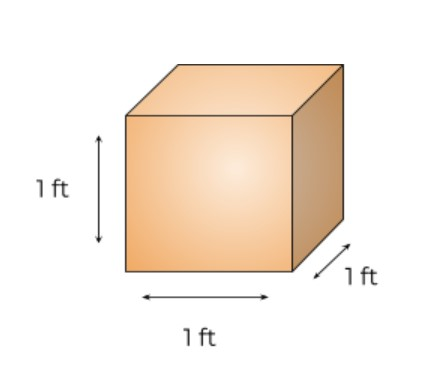# Cubic Feet Calculator

Enter the length, width, and height of the object in the Cubic feet Calculator to find its volume.The cubic foot (cft) calculator is an online tool for computing the volume of a material or space. This tool is one of the best because it has the following features:

• Users can enter values in different units of measurements like inches, meters e.t.c.
• Full calculation process that first finds the volume in the inputted unit and then converts it into the cubic feet.
• It gives a labeled diagram of the cube that has a geometry according to the entered values.

## How to use this calculator?

All you have to do is:

1. Enter lengths.
2. Choose the unit of the values inputted.
3. Click “Calculate”.

Keep reading to know more about cubic feet, its formula, and how to figure cubic feet manually.

## What is a cubic foot?

It is a unit of volume. A cubic foot is not related to cubes only but it actually means what is the volume of the body in terms of a cube that is 1 foot tall, long, and wide.For example, if you want to find out the volume of cement in cubic feet, it will be the volume of cement that can fit into the cubic cylinder of volume 1ft3 (as shown in the image).

## Cubic feet formula

The formula to find the volume in cubic feet is the same as volume. Just make sure your values are in feet.

Volume in cft = Length (ft) x Width (ft) x height (ft)

Note that to use this formula, the body must have noticeable length, width, and height unlike spheres, cones.

For such bodies, you convert the measurements in feet and then find the volume using their specified volume formulas.

## How to calculate cubic feet?

To find the volume in the cubic feet yourself, you will need to follow the instructions below.

1. Measure the height, length, and width of the object. For materials like cement or mud, measure the cylinder which is holding them.
2. Convert the values in feet.
3. Multiply the three values.

And just like that, you have the volume in cft. Maybe confirm your answer from the cubic ft calculator afterward.

Example:

Find the volume of a rectangle in cubic feet of a cylinder with the following dimensions.

Length = 20 meters

Height = 15 meters

Width = 20 meters

Solution:

By using the usual volume formula, you will get the result in cubic meters. So to calculate in cubic feet.

Step 1: Convert the meters to feet.

As 1 meter = 3.28084 feet

Length = 20 m = 20 x 3.28084 feet

= 65.17 feet

Height = 15 m = 15 x 3.28084 feet

= 49.213 feet

Width = 20 m = 20 x 3.28084 feet

= 65.17 feet

Step 2: Multiply the values according to the cubic feet formula.

= L x H x W

= 65.17 x 49.213 x 65.17

= 211888 ft

## Calculating cubic feet from other units

To find the volume in cubic feet when the measured lengths are in other units, you can use the following formulas.

From inches:

Divide each length by 12.

From yards:

Multiply each length by 3.

From meters:

Multiply by 3.28084

From millimeters:

Multiply by 0.00328

From centimeters:

Multiply each value by 0.0328084